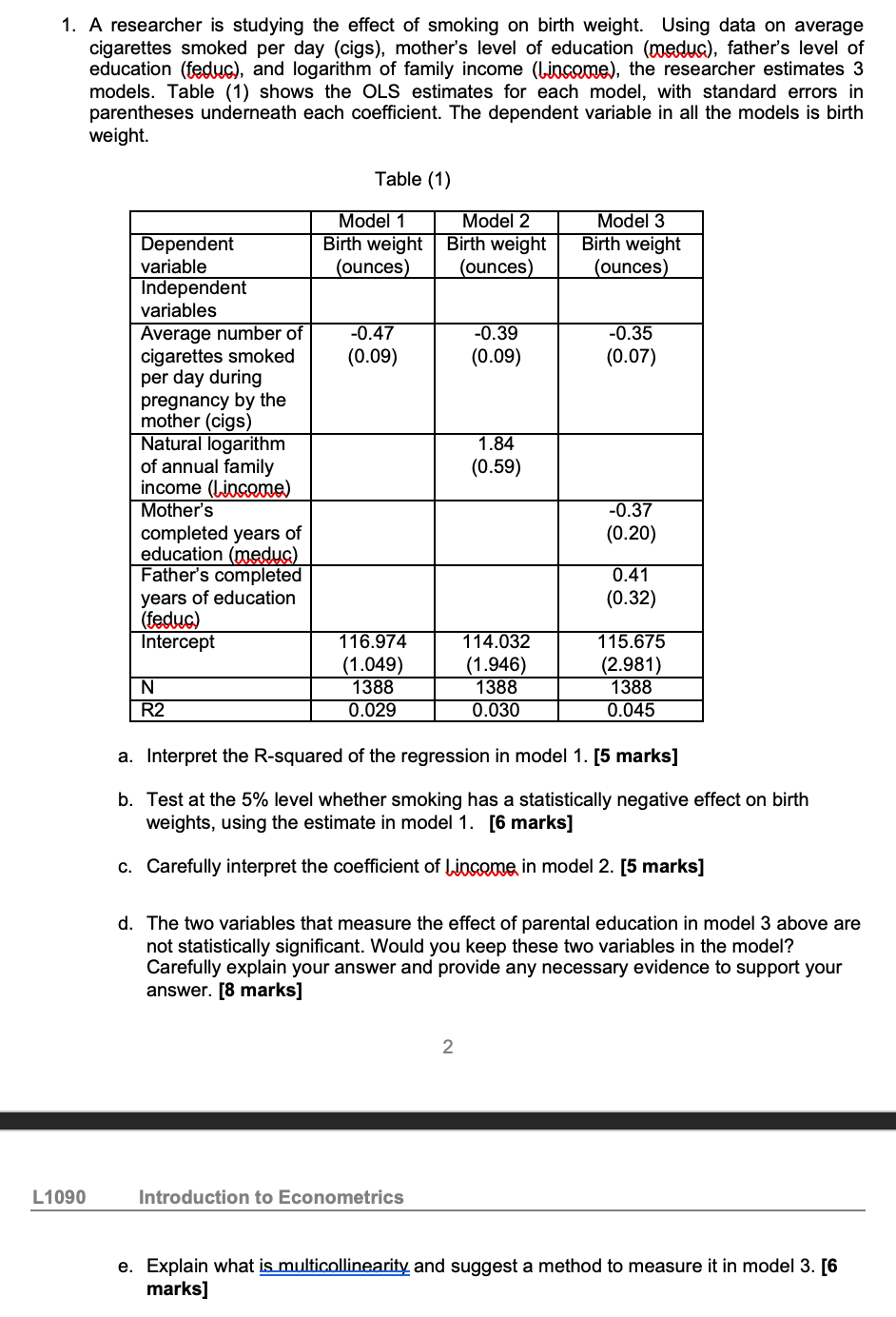# (Solved): please answer all questions 1. A researcher is studying the effect of smokin ...???????

1. A researcher is studying the effect of smoking on birth weight. Using data on average cigarettes smoked per day (cigs), mother's level of education (meduc), father's level of education (feduc), and logarithm of family income (Lincome), the researcher estimates 3 models. Table (1) shows the OLS estimates for each model, with standard errors in parentheses underneath each coefficient. The dependent variable in all the models is birth weight. Table (1) a. Interpret the R-squared of the regression in model 1. [5 marks] b. Test at the \( 5 \% \) level whether smoking has a statistically negative effect on birth weights, using the estimate in model 1. [6 marks] c. Carefully interpret the coefficient of Lircome in model 2. [5 marks] d. The two variables that measure the effect of parental education in model 3 above are not statistically significant. Would you keep these two variables in the model? Carefully explain your answer and provide any necessary evidence to support your answer. [8 marks] 2 Introduction to Econometrics e. Explain what is multicollinearity and suggest a method to measure it in model 3. [6 marks]

We have an Answer from Expert